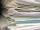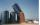Homework

Mother and Kate together weigh 24.5 kg more than the father. Father weighs 7.6 kg more than mother. Father weight 44.8 kg more than Kate. Determine weights of father mother and Kate.

Result

k =  32.1
m =  69.3
o =  76.9

Solution:

m+k=o+24.5
o = m + 7.6
o = k + 44.8

k+m-o = 24.5
m-o = -7.6
k-o = -44.8

k = 32110 = 32.1
m = 69310 = 69.3
o = 76910 = 76.9

Calculated by our linear equations calculator.

Leave us a comment of example and its solution (i.e. if it is still somewhat unclear...):Be the first to comment!To solve this example are needed these knowledge from mathematics:

Do you have a linear equation or system of equations and looking for its solution? Or do you have quadratic equation?

Next similar examples:

1. Iron collectingClass 7A collected 3.2 tonnes of iron more than class 7B. Together they collected 6.4 tonnes of iron to the secondary raw material collection. How much did each class collect?
2. Waiting roomIn the waiting room are people and flies. Together they have 15 heads and 50 legs (fly has 6 legs). How many people and flies are in the waiting room?
3. Barrel 4Barrel of water weighs 63 kg. After off 75% water, the weight of the barrel with water is 21 kg. How many kg weigh empty barrel and how many kgs water in it?
4. SchoolsThree schools are attended by 678 pupils. To the first attend 21 students more and to the third 108 fewer students than to second school. How many students attend the schools?
5. Two trainsThere were 159 freight wagons on the railway station creating 2 trains. One had 15 more wagons than the other. How many wagons did each train have?
6. Three brothersThe three brothers have a total of 42 years. Jan is five years younger than Peter and Peter is 2 years younger than Michael. How many years has each of them?
7. Three figures - numbersThe sum of three numbers, if each is 10% larger than the previous one, is 662. Determine the figures.
8. Equation?
9. Equation 29Solve next equation: 2 ( 2x + 3 ) = 8 ( 1 - x) -5 ( x -2 )
10. Bakery and flourThe bakery tray for flour was filled to 3/4 volume. After removing 875 kg of flour, it was filled to only 2/5 of the volume. How many tons of flour is in the full tray?
11. Simple equationSolve the following simple equation: 2. (4x + 3) = 2-5. (1-x)
12. Dropped sheetsThree consecutive sheets dropped from the book. The sum of the numbers on the pages of the dropped sheets is 273. What number has the last page of the dropped sheets?
13. Spain vs USASpain lost to the US by 4 goals. In the match total fell 10 goals. How many goals gave the Spain and how the United States?
14. Find xSolve: if 2(x-1)=14, then x= (solve an equation with one unknown)
15. Forest nurseryIn the forest nursery after winter, they found that 1/10 stems died out of them. For them, they land 193 new spruces. How many spruces are in the forest nursery?
16. ATC campThe owner of the campsite offers 79 places in 22 cabins. How many of them are triple and quadruple?
17. 13 ticketsA short and long sightseeing tour is possible at the castle. Ticket for a short sightseeing circuit costs CZK 60, for a long touring circuit costs CZK 100. So far, 13 tickets have been sold for 1140 CZK. How much did you pay for tickets for a short tour?